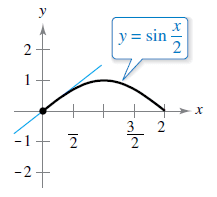### Create an Account

Home / Questions / Slope of a Tangent Line In Exercises 61 and 62, find the slope of the tangent line to the ...

# Slope of a Tangent Line In Exercises 61 and 62, find the slope of the tangent line to the sine function at the origin. Compare this value with the number of complete cycles in the interval . Chapter

Slope of a Tangent Line In Exercises 61 and 62, find the slope of the tangent line to the sine function at the origin. Compare this value with the number of complete cycles in the interval.Jul 30 2021 View more View LessSubscribe To Get Solution